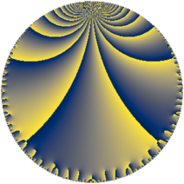# Properties

 Label 38.6.eLevel $38$ Weight $6$ Character orbit 38.e Rep. character $\chi_{38}(5,\cdot)$ Character field $\Q(\zeta_{9})$ Dimension $54$ Newform subspaces $2$ Sturm bound $30$ Trace bound $1$

# Related objects

## Defining parameters

 Level: $$N$$ $$=$$ $$38 = 2 \cdot 19$$ Weight: $$k$$ $$=$$ $$6$$ Character orbit: $$[\chi]$$ $$=$$ 38.e (of order $$9$$ and degree $$6$$) Character conductor: $$\operatorname{cond}(\chi)$$ $$=$$ $$19$$ Character field: $$\Q(\zeta_{9})$$ Newform subspaces: $$2$$ Sturm bound: $$30$$ Trace bound: $$1$$ Distinguishing $$T_p$$: $$3$$

## Dimensions

The following table gives the dimensions of various subspaces of $$M_{6}(38, [\chi])$$.

Total New Old
Modular forms 162 54 108
Cusp forms 138 54 84
Eisenstein series 24 0 24

## Trace form

 $$54q + 33q^{3} - 12q^{6} + 354q^{7} - 192q^{8} + 33q^{9} + O(q^{10})$$ $$54q + 33q^{3} - 12q^{6} + 354q^{7} - 192q^{8} + 33q^{9} + 474q^{11} - 4272q^{13} - 2640q^{14} - 6366q^{15} + 6096q^{17} + 12648q^{18} + 7170q^{19} + 2112q^{20} - 3996q^{21} - 4944q^{22} - 4476q^{23} - 192q^{24} - 12120q^{25} - 1320q^{26} + 7887q^{27} + 6816q^{28} - 1566q^{29} - 6546q^{31} + 5955q^{33} - 9168q^{34} + 46524q^{35} + 528q^{36} + 20052q^{37} - 6756q^{38} - 28956q^{39} - 3249q^{41} + 17712q^{42} + 16890q^{43} + 21504q^{44} - 66114q^{45} - 45600q^{46} - 136794q^{47} - 16896q^{48} - 62355q^{49} - 90996q^{50} + 48009q^{51} + 3936q^{52} + 9180q^{53} + 138420q^{54} + 100458q^{55} + 66816q^{56} + 411072q^{57} + 64416q^{58} + 292569q^{59} - 9696q^{60} - 87474q^{61} - 43272q^{62} - 358188q^{63} - 110592q^{64} - 283320q^{65} - 258612q^{66} - 385341q^{67} - 30384q^{68} - 144162q^{69} + 9168q^{70} + 493638q^{71} + 92544q^{72} + 411954q^{73} - 111240q^{74} + 37920q^{76} + 127380q^{77} + 437928q^{78} + 12318q^{79} - 32469q^{81} - 311220q^{82} - 350070q^{83} - 231264q^{84} - 273264q^{85} - 133248q^{86} - 193638q^{87} - 46464q^{88} + 94464q^{89} + 609456q^{90} - 407580q^{91} + 358368q^{92} + 574278q^{93} + 149328q^{94} + 1342920q^{95} + 749811q^{97} + 134784q^{98} - 412095q^{99} + O(q^{100})$$

## Decomposition of $$S_{6}^{\mathrm{new}}(38, [\chi])$$ into newform subspaces

Label Dim. $$A$$ Field CM Traces $q$-expansion
$$a_2$$ $$a_3$$ $$a_5$$ $$a_7$$
38.6.e.a $$24$$ $$6.095$$ None $$0$$ $$18$$ $$0$$ $$438$$
38.6.e.b $$30$$ $$6.095$$ None $$0$$ $$15$$ $$0$$ $$-84$$

## Decomposition of $$S_{6}^{\mathrm{old}}(38, [\chi])$$ into lower level spaces

$$S_{6}^{\mathrm{old}}(38, [\chi]) \cong$$ $$S_{6}^{\mathrm{new}}(19, [\chi])$$$$^{\oplus 2}$$